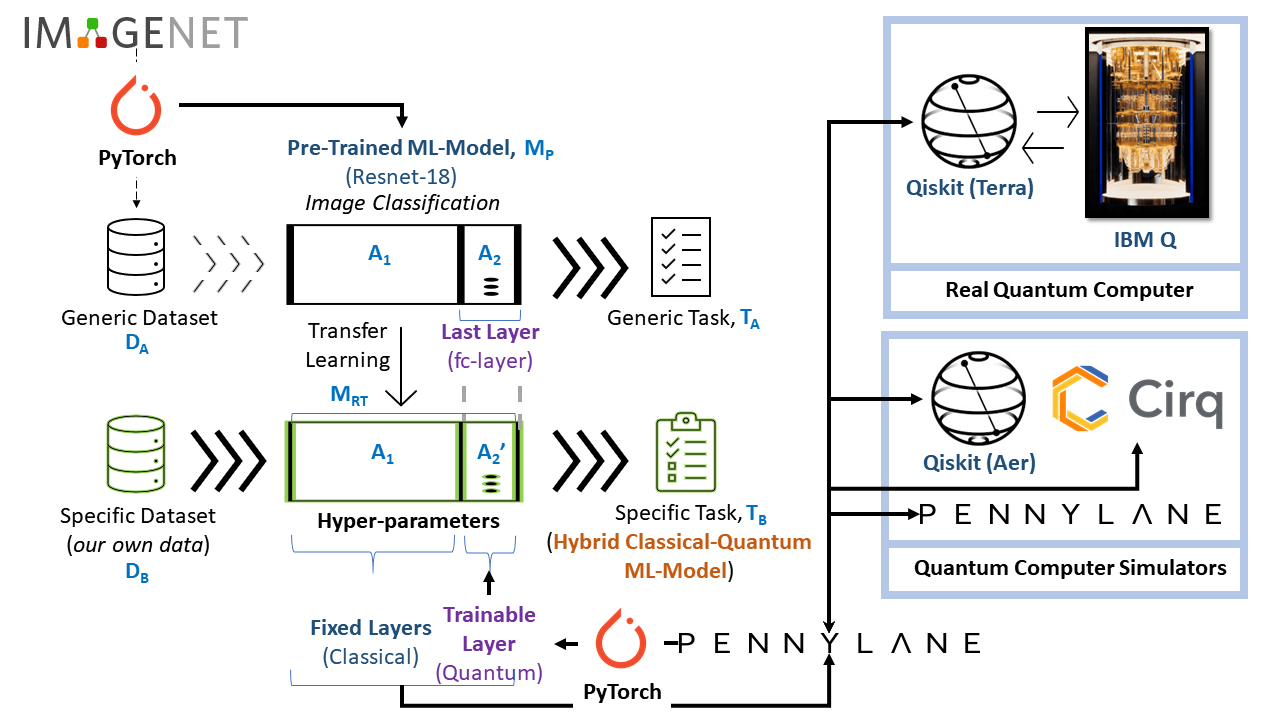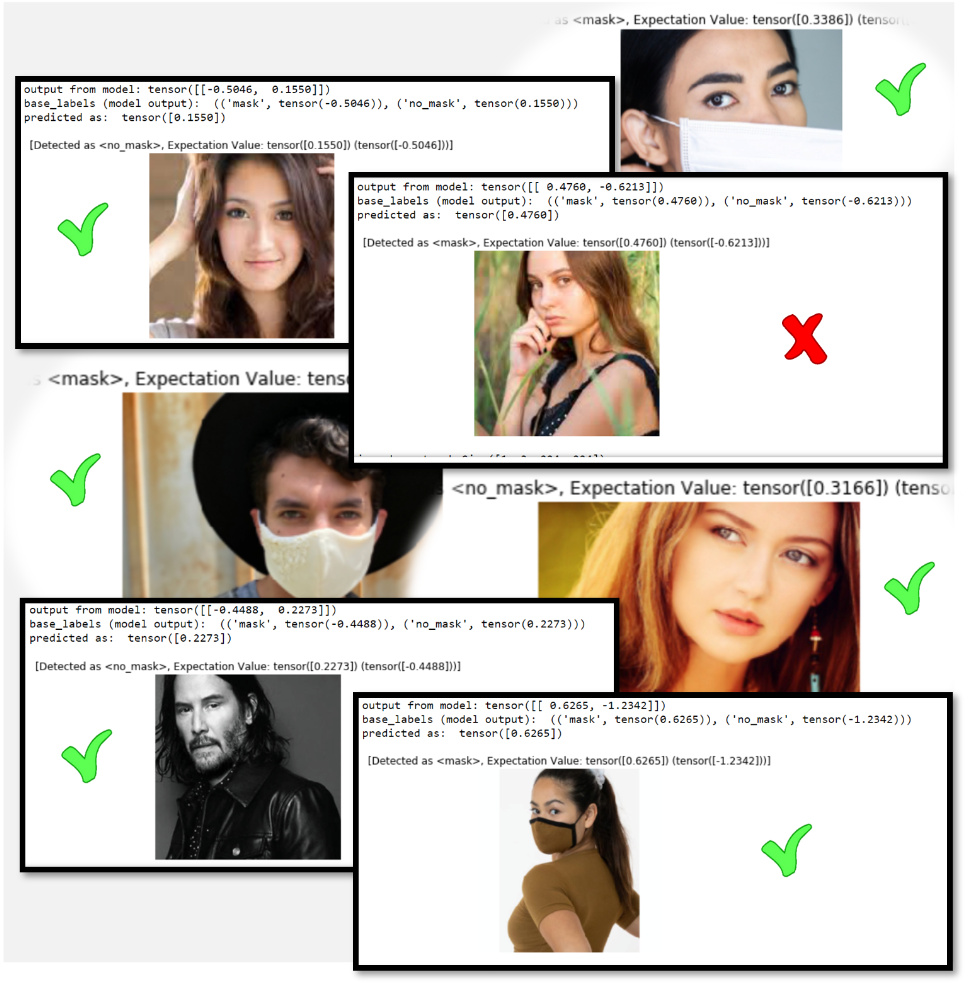# Hello Tomorrow — I am a Hybrid Quantum Machine Learning

`*** Supporting files (Python Notebook, images) for this article are available in github.*** Articles on General Introduction to Quantum Computing are available in "Meneropong Masa Depan: Quantum Computing" (Indonesian), "The Race in Achieving Quantum Supremacy & Quantum Advantage" (English), "Hello Many Worlds in Quantum Computer" (English), "Quantum Teleportation" (English), and "Quantum Random Number Generator"(English).`A generic neural network model (Machine Learning model) trained on the ImageNet dataset is used as the base for Transfer Learning on the Image Classification task (based on ResNet-18 neural network architecture). The last layer of this pre-trained model (fully-connected layer) is modified by quantum through a quantum machine learning framework: Pennylane.ai. The framework (which is open-source) provides convenient access to multiple quantum simulators and real quantum computer backend, including IBM real Quantum Computer on IBM Quantum Computing Experience (IBM Cloud) through an open-source Qiskit API (Application Programming Interface) accessible by Python programming language.

When we talk about quantum computers, we usually mean fault-tolerant devices.” (James Wootton, 2018). James continues, “We’ll know that devices can do things that classical computers can’t, but they won’t be big enough to provide fault-tolerant implementations of the algorithms we know about. John Preskill coined the term NISQ to describe this era. Noisy because we don’t have enough qubits to spare for error correction, and so we’ll need to directly use the imperfect qubits at the physical layer. And ‘Intermediate-Scale’ because of their small (but not too small) qubit number.

# 2. Building a Hybrid Classical-Quantum Machine Learning ModelThe possible combinations of processing data and algorithms by classical and/or quantum computers. CQ (processing data in a classical computer and algorithm in a quantum computer) is the combination discussed in this article.
`# 1st - Prepare the Quantum Gatesdef H_layer(nqubits):    """Layer of single-qubit Hadamard gates.    """    for idx in range(nqubits):        qml.Hadamard(wires=idx)        def RY_layer(w):    """Layer of parametrized qubit rotations around the y axis.    """    for idx, element in enumerate(w):        qml.RY(element, wires=idx)        def entangling_layer(nqubits):    """Layer of CNOTs followed by another shifted layer of CNOT.    """    # In other words it should apply something like :    # CNOT  CNOT  CNOT  CNOT...  CNOT    #   CNOT  CNOT  CNOT...  CNOT    for i in range(0, nqubits - 1, 2):  # Loop over even indices: i=0,2,...N-2        qml.CNOT(wires=[i, i + 1])    for i in range(1, nqubits - 1, 2):  # Loop over odd indices:  i=1,3,...N-3        qml.CNOT(wires=[i, i + 1])@qml.qnode(dev, interface="torch")def quantum_net(q_input_features, q_weights_flat):    """    The variational quantum circuit.    """    # Reshape weights    q_weights = q_weights_flat.reshape(q_depth, n_qubits)# Start from state |+> , unbiased w.r.t. |0> and |1>    H_layer(n_qubits)        # Embed features in the quantum node    RY_layer(q_input_features)        # Sequence of trainable variational layers    for k in range(q_depth):        entangling_layer(n_qubits)        RY_layer(q_weights[k])            # Expectation values in the Z basis    exp_vals = [qml.expval(qml.PauliZ(position)) for position in range(n_qubits)]    return tuple(exp_vals)`
`# 2nd - Prepare the Replacement Quantum Layerclass DressedQuantumNet(nn.Module):def __init__(self):        super().__init__()        self.pre_net = nn.Linear(512, n_qubits)        self.q_params = nn.Parameter(q_delta * torch.randn(q_depth * n_qubits))        self.post_net = nn.Linear(n_qubits, 2)def forward(self, input_features):        # obtain the input features for the quantum circuit        # by reducing the feature dimension from 512 to 4        pre_out = self.pre_net(input_features)        q_in = torch.tanh(pre_out) * np.pi / 2.0# Apply the quantum circuit to each element of the batch and append to q_out        q_out = torch.Tensor(0, n_qubits)        q_out = q_out.to(device)        for elem in q_in:            q_out_elem = quantum_net(elem, self.q_params).float().unsqueeze(0)            q_out = torch.cat((q_out, q_out_elem))# return the two-dimensional prediction from the postprocessing layer        return self.post_net(q_out)# 3rd - Replace last layer of ResNet-18 with defined quantum layermessage = "* Loading pre-trained ResNet-18..."myprint(log_file_name, "append", message, screen=True)model_hybrid = torchvision.models.ResNet18(pretrained=True)for param in model_hybrid.parameters():    param.requires_grad = False# Notice that model_hybrid.fc is the last layer of ResNet18message = "  - Replacing last layer (fc-layer) with Quantum Layer..."myprint(log_file_name, "append", message, screen=True)model_hybrid.fc = DressedQuantumNet()# Use CUDA or CPU according to the "device" object.model_hybrid = model_hybrid.to(device)`
`* (START re-training the Quantum Layer)  => Training Started.     > Phase: train Epoch: 1/30 Loss: 0.6145 Acc: 0.6875             > Phase: validation   Epoch: 1/30 Loss: 0.4807 Acc: 0.8400        * Saving interim model while training: swgCQ_simIBMQLocal-at-epoch-1(30)-14072020172453.pth[[1.         0.6875     0.6144937  0.84       0.48068776]]     > Phase: train Epoch: 2/30 Loss: 0.4623 Acc: 0.8125             > Phase: validation   Epoch: 2/30 Loss: 0.3722 Acc: 0.9200        * Saving interim model while training: swgCQ_simIBMQLocal-at-epoch-2(30)-14072020172453.pth[[2.         0.8125     0.4623062  0.92       0.37219827]]     > Phase: train Epoch: 3/30 Loss: 0.3748 Acc: 0.9125             > Phase: validation   Epoch: 3/30 Loss: 0.2874 Acc: 0.9800        * Saving interim model while training: swgCQ_simIBMQLocal-at-epoch-3(30)-14072020172453.pth[[3.         0.9125     0.37478789 0.98       0.28741294]]     > Phase: train Epoch: 4/30 Loss: 0.3427 Acc: 0.9042             > Phase: validation   Epoch: 4/30 Loss: 0.2684 Acc: 0.9800        * Saving interim model while training: swgCQ_simIBMQLocal-at-epoch-4(30)-14072020172453.pth.........      > Phase: train Epoch: 29/30 Loss: 0.2568 Acc: 0.9292             > Phase: validation   Epoch: 29/30 Loss: 0.1558 Acc: 1.0000        * Saving interim model while training: swgCQ_simIBMQLocal-at-epoch-29(30)-14072020172453.pth[[29.          0.97083333  0.18603694  1.          0.14358226]]     > Phase: train Epoch: 30/30 Loss: 0.1930 Acc: 0.9708             > Phase: validation   Epoch: 30/30 Loss: 0.1495 Acc: 1.0000        * Saving interim model while training: swgCQ_simIBMQLocal-at-epoch-30(30)-14072020172453.pth[[30.          0.97083333  0.18603694  1.          0.14358226]]* Saving train, val results (all epochs): 'epoch, best_acc_train, best_loss_train, best_acc, best_loss'  => Training completed in 219m 52s  => Best test loss: 0.1436 | Best test accuracy: 1.0000* (FINISH re-training the Quantum Layer).`
`hybrid_model_name = "swgCQ_simIBMQLocal(30)-08072020085403.pth"my_hybrid_model_name = hybrid_model_namemy_model_hybrid = torchvision.models.ResNet18(pretrained=True)for param in my_model_hybrid.parameters():    param.requires_grad = Falsemy_model_hybrid.fc = DressedQuantumNet()my_model_hybrid = my_model_hybrid.to(device)my_model = my_model_hybridmy_model.load_state_dict(torch.load(my_hybrid_model_name))#  Predict a single imagefrom PIL import Imagedata_transforms = transforms.Compose([            transforms.Resize(256),            transforms.CenterCrop(224),            transforms.ToTensor(),            transforms.Normalize([0.485, 0.456, 0.406], [0.229, 0.224, 0.225]),        ])def predict_single_image(model, image):    PIL_img = Image.open(image)    img = data_transforms(PIL_img)    # add 1 dimension at position 0    img_input = img.unsqueeze(0)     print("img shape", img_input.shape)    print(type(img_input))       model.eval()    with torch.no_grad(): # inferencing        outputs = model(img_input)        print("output from model:", outputs)        base_labels = (("mask", outputs[0,0]), ("no_mask", outputs[0,1]))        expvals, preds = torch.max(outputs, 1)        expvals_min, preds_min = torch.min(outputs, 1)        print("base_labels (model output): ", base_labels)        print("predicted as: ", expvals)         if expvals == base_labels:            labels = base_labels        else:            labels = base_labels        ax = plt.subplot()        ax.axis("off")        title = "Detected as <" + labels + ">, Expectation Value: " + str(expvals) + " (" + str(expvals_min) + ")"         ax.set_title("[{}]".format(title))        imshow(img)`A few examples of prediction during inference using trained hybrid classical-quantum machine learning model ‘swgCQ_simIBMQLocal(30)-08072020085403.pth’ applied test dataset. Note that predictions are not 100% accurate.

--

--